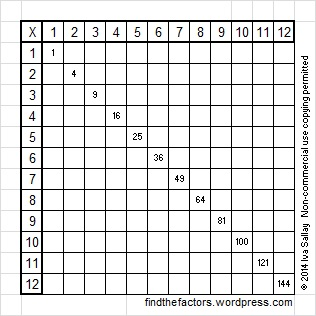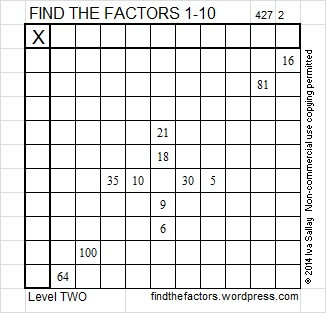# Puzzle Levels Explained

The object of the FIND THE FACTORS 1 – 10 (or 1 – 12) puzzle is to write the numbers 1 to 10 (or 1 to 12) in the top row and again in the first column so that once the factors are found, the puzzle works as a multiplication table. All of the puzzles require a basic, but not necessarily quick or perfect, knowledge of all the multiplication facts from 1 to 10 or from 1 to 12. Each puzzle has only one solution, and it can be found using logic. Even though some of these puzzles can be completed by 3rd grade students, I know adults who have math or science related degrees who also solve them regularly regardless of the level of the puzzle. Once a level has been mastered, work on mastering the next level as well. Within each level some puzzles might be more difficult than others for a person to complete. In general the FIND THE FACTORS 1 – 12  puzzles are more difficult to complete than the puzzles that use only multiplication facts up to 100.Level ONE puzzles allow a person with only a limited understanding of division or factoring to solve and complete a puzzle. Each puzzle concentrates on up to 12 division facts: If the only division facts a person knows is how to divide by 2, there is a puzzle where all the clues are divisible by 2. When that person later learns how to divide by 5, there is a puzzle that focuses only on those division facts. These puzzles are ideal for those just learning division while all the multiplication facts are reinforced as well.The fact that one row and one column contain no clues can be confusing the first time a person does one of these puzzles. The factor for that row or that column can be deduced by completing the rest of the puzzle and simply counting from 1 to 10 (or 1 to 12) and determining which number is missing from that row or column.

In a classroom setting a teacher may inform the students what number will factor into every clue, but when that information is not given, the puzzles are a little more challenging. So that teachers do not have to search the blog for specific puzzles, I’ve included an excel file where each number from 1 to 12 is the common factor of its own puzzle here: Division Facts Puzzles. The factor answers are typed in light blue that should become invisible when copied on a copy machine.

Sometimes all the clues in a level ONE puzzle are perfect squares. These puzzles can be introduced once a person notices the special numbers that appear on the diagonal of a regular multiplication table. Most products appear at least two times on a multiplication table, but perfect squares 1, 25, 49, 64, 81, 100, 121, and 144 each appear only once.When perfect squares are used as clues in the FIND THE FACTORS puzzles, one of the perfect squares will be left out, but it is easy to use logic to determine what that perfect square should be. Thus it is possible to complete an entire 10 x 10 puzzle when only 9 clues are given or a 12 x 12 puzzle when only 11 clues are given.

When I first created these factoring puzzles in 1997, a puzzle with only perfect square clues was the first one that I made. All the rest of the puzzles I made at that time were what I now call level FOUR puzzles. I didn’t make any of the other types of level one puzzles until 2013 when I began this blog. Jumping from Level ONE to Level FOUR was quite a leap for some people, so in 2011 I created the first Level TWO puzzle.Level TWO puzzles require familiarity with all the facts in a multiplication table. Any clue that is not a perfect square will have at least 2 other clues in the same row or column, and there will always be only one common factor for those clues. If a factor is chosen that is the common factor of all but one of the clues, the mistake should be caught rather quickly. For example if clues 8, 16, 32, and 36 all appeared in the same row, a person might initially think 8 is the common factor, but as the first row gets filled in, the fact that 36 is a multiple of 4 but of 8 will most likely get noticed.  That is as tricky as a level 2 puzzle will get.

Level THREE puzzles were first designed in 2013 a month before I began this blog when I noticed some people struggling to make the leap from level TWO to what is now Level FOUR. In a level THREE puzzle there will always be a column or a row with two clues. When all the factors from 1 to 10 (or 1 to 12) are considered there will always be exactly one common factor that will work with those two clues. When the factors are written or typed into the corresponding cells, the top cell of the first column will always have a factor written in it and there will be two other factors written in either the factor column or the factor row. One of those factors written will always be the needed factor that will go into the clue in the next row. Sometimes the third factor will be picked up somewhere in the middle of the puzzle, while this process of working from the top to the bottom continues until the puzzle is completed. Once I did publish a Level THREE  puzzle that had to be completed from the bottom up, but in all other cases Level THREE puzzles are worked from the top to the bottom of the first column. In this Level THREE puzzle 20, 8, 2, and 18 are all multiples of 2, but as the puzzle is worked from top to bottom, all but two of those clues will be eliminated as we find the multiples of 4 and 8. Likewise 18, 21, & 27 are all multiples of 3, and 60, 66, & 18 are all multiples of 6, and 44, 20, 40, & 8 are all multiples of 4. In every case as this puzzle is worked from top to bottom, we are able to rely on this feature and less on logic to find the puzzles unique solution.Level FOUR puzzles are similar to Level THREE puzzles except there is no restriction on which order the clues are written or the factors are found. When I first began this blog I published a few level THREE puzzles and a few level FOUR puzzles that had exactly the same clues. If you compare the puzzle below with the Level THREE puzzle above you will notice that the clues are exactly the same but in different places. This level FOUR  puzzle is solved using the clues in the exact same order as they were used in the level THREE  puzzle, but now using logic is so necessary to help us know if 20 is 2 x 10 in the puzzle or if it is 4 x 5 and so forth with several other of the clues.Level FOUR puzzles will have at least one row or column with more than one clue, but in EVERY case, those clues will have only one common factor.

Level FIVE puzzles are like level FOUR puzzles, but there will be at least two rows or columns with multiple clues. There will be rows or columns that have clues that have more than one common factor, but there will always be at least one row or column with multiple clues that has only one common factor. Those are the clues with which to begin as they will help get the puzzle solved with the least amount of erasing.• In this Level 5 puzzle 3 and 9 have two common factors: 1 and 3.
• Likewise 20 and 40 have three common factors that potentially could work in the puzzle: 4, 5 or 10.
• 12 and 30 have 2 possible common factors: 3 or 6.
• But 40 and 90 have only one possible common factor: 10, so that’s the best place to begin.

Level SIX puzzles have rows or columns with multiple clues, and in every case, the clues have more than one common factor. It may be the first common factor that pops in your mind, but it may not be. These puzzles can still be solved using only logic, but it is trickier. I strongly suggest using logic instead of guess and check to solve these puzzles.Solutions for the puzzles are often given a week or two after a puzzle is initially published on the same blog post where it was originally published. In the case of Level 4, 5, and 6 puzzles, instead of giving the actual solution, I usually give a chart showing the logical order to arrive at it. If you start at the top of this chart and fill in the answers to the Level SIX  puzzle above, you will arrive at the solution logically. There can be more than one way to arrive at the solution. The chart maps out one of those ways. It also explains why some factors work while others don’t. Sometimes I use short, but confusing sentences to explain. In the chart below I’m trying to say that 5, 10, & 11 must appear in both the 1st column and the top row. Since only one clue is a multiple of 11, it must be the factor that goes in either the column or row that doesn’t contain a clue which means 5 & 10 cannot be the factors that go into both of the empty column and empty row. 11 has to go into one of them. The only way that can happen is if 6 is the common factor for 30 and 60. Perhaps the long explanation is just as confusing as the short one, but if you follow these steps, you will be able to solve the level SIX puzzle.This site uses Akismet to reduce spam. Learn how your comment data is processed.